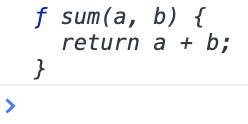# JavaScript Functions Interview Questions

Last updated on 14 Nov, 2020

Question:

How are functions stored in memory by JavaScript? Here we have a function `sum()`.

``````function sum(a, b) {
return a + b;
}``````

How `sum()` function is stored in memory?

JavaScript allocates a memory block, just like a memory is allocated for a variable. The definition of the function is then stored inside the variable. That is why if we print the value of `sum` in console, it prints out the full source code of the function.

``console.log(sum);``

Here is the console output:----o----

Question:

Does a function always return a value?

Yes. If there is a `return` statement, that value is returned. If there is no `return` statement, the function implicitly returns `undefined`.

Here is a function that explicitly returns a number.

``````function f() {
return 7;
}
const result = f();
console.log(result); // 7``````

Here is a function that does not have `return` statement.

``````function f() {
var a = 7;
}
const result = f();
console.log(result); // undefined``````
--- ○ ---